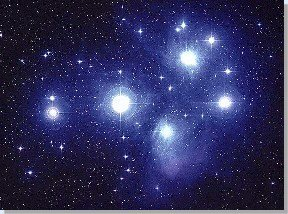Infinite Velocity and FTL Phenomena
(c) Robert Neil BoydSuperluminal and Subluminal Signals (R. M. Kiehn) -- The non-linear Moebius projective transformation fractional solutions to the Maxwell equations allow that the propagation speed of the E/M singularity can be anything - including infinity!

Segal's Conformal Theory (Tony Smith) -- The 4 GraviPhoton Special Conformal transformations are like the Moebius linear fractional transformations, and are the symmetries of superluminal solutions of the Maxwell equations.

Publications and Abstracts:

Experimental Evidence of Near-Field Superluminally Propagating Electromagnetic Fields (William Walker)

Faster Than Light? (Rodrigues, Maiorino)

Geometry of spacetime with superluminal phenomena (Rodrigues, Matolcsi)

Launching of Non-Dispersive Superluminal Beams (Rodrigues, Barashenkov)

Lawbreakers? The physics of superluminal sources (Singleton, John) -- The emission of electromagnetic radiation from a superluminal (faster-than-light in vacuo) charged particle was first studied by Sommerfeld in 1904. However, the Special Theory of Relativity was published just a few months later; prevailing scientific opinion then effectively curtailed the research field until Ginzberg and coworkers pointed out in the 1980s that no physical principle forbids emission by extended, massless superluminal sources. A displacement current density (dD/dt; see Maxwell's fourth equation) can provide such a source; the individual charged particles creating the polarization do not move faster than the speed of light, and yet it is relatively trivial to make the envelope of the displacement current density to do so.

Maxwell and Dirac theories as an already unified theory (Rodrigues, Vaz) -- In this paper we formulate Maxwell and Dirac theories as an already unified theory (in the sense of Misner and Wheeler). We introduce Dirac spinors as "Dirac square root" of the Faraday bivector, and use this in order to find a spinorial representation of Maxwell equations. Then we show that under certain circumstances this spinor equation reduces to an equation formally identical to Dirac equation. Finally we discuss certain conditions under which this equation can be really interpreted as Dirac equation, and some other possible interpretations of this result.

On the Equation rotA = K A (Rodrigues, Vaz)

On the Existence of Undistorted Progressive Waves (UPWs) of Arbitrary Speeds $0 \leq v< \infty$ in Nature
(Rodrigues, Lu)

Smarandache Hypothesis: Evidences, Implications and Applications (Leonardo F. D. da Motta) -- Note: To view the original Smarandache Hypothesis, please refer to http://www.gallup.unm.edu/~smarandache/NoSpLim.htm

Spacetime model with superluminal phenomena (Rodrigues, Vaz)

Subluminal and Superluminal Electromagnetic Waves and the Lepton Mass Spectrum (Rodrigues, Vaz)

Subluminal and superluminal solutions in vacuum of the Maxwell equations and the massless Dirac equation (Rodrigues, Vaz)

Superluminal Near-Field Dipole Electromagnetic Fields (William D. Walker)

Superluminal Ultrasound? -- The American Institute of Physics Bulletin of Physics News Number 751 October 26, 2005 by Phillip F. Schewe, Ben Stein

The X-shaped, localized field generated by a Superluminal electric charge (Erasmo Recami, M. Zamboni-Rached, Cesar A. Dartora, K. Z. Nobrega)

Unified theory for construction of arbitrary speeds (0 < = v < \infty) solutions of the relativistic wave equations (Rodrigues, Maiorino) -- Representing the relativistic physical fields as sections of the Clifford Bundle (or of the Spin-Clifford Bundle) of Minkowski spacetime we show that all the relativistic wave equations satisfied by these fields possess solutions traveling with arbitrary speeds $0 \leq v < \infty$. By giving rigorous mathematical definitions of reference frames and of the Principle of Relativity (PR) we prove that physical realizations of the $v > 1$ solutions of, e.g., the Maxwell equations imply in a breakdown of the PR, but in no contradiction at all with known physical facts.